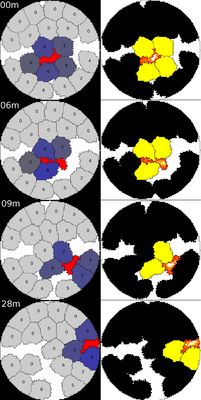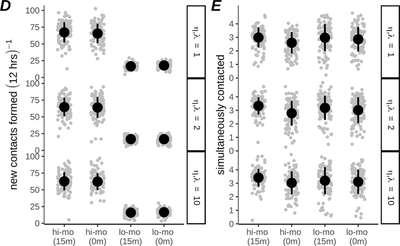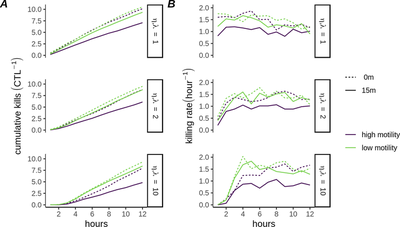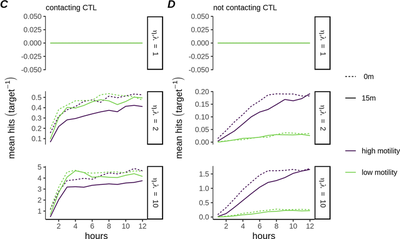# Spatial Effects on Killing Kinetics of Cytotoxic T Lymphocytes

##### Persistent Identifier

This model also requires the separate file boundary.tiff.

## Introduction

The immune system plays an important role in controlling infections and tumors. Knowledge about the mechanisms through which the immune system accomplishes this can be exploited to develop immunotherapies. A pivotal mechanism involves killing of target cells by Cytotoxic T Lymphocytes (CTLs).

A major limitation of many prior estimates of CTL killing is that analysis is performed on population level data in in vivo settings, with no direct measurements of the killing process. This approach has a number of drawbacks:

1. It can be challenging to accurately assess the frequency of CTLs and target cells.
2. Other immune cells may contribute to the killing process, confounding estimates of the true CTL killing rate.
3. The processes underlying CTL killing are complex and it may be insufficient to describe them with a single, time invariant rate constant.

Besides analysing CTL killing performance at the population level, a potentially useful approach is to analyse CTL killing at the single cell level. Such analysis can yield greater insights into the dynamics of the killing process.

Here, stochastic simulations of single CTLs killing small populations of target cells were developed, showing that multiple-hitting can lead to apparently heterogeneous killing kinetics between otherwise identical CTLs.

## Description

An agent based cellular Potts model (CPM) was employed to generate 2D simulations of CTLs interacting with and killing targets over a period of 12 hours (StopTime = 43200 s). For all CPM simulations the same underlying gamma model of CTL hit generation was maintained as was used for the Monte-Carlo simulations, however several modifications were made that would lead to different (yet not predictable a priori) distributions of hits amongst targets:

• Specifically, instead of allocating hits to all contacted targets with equal probability, target risk of receiving a hit was proportional to the length of the interface between CTL and target ctl.contact.length at the moment of hit generation.
• We also considered the effect of a lower bound on the time (time-step = 60 s with trigger = when-true) required for a CTL to complete a hit, by introducing a delay condition maturity > minhit that prohibited targets from being hit within an initial time window after contacting a CTL, which was reset (immaturing Event) every time the target broke contact with the CTL. Note that the delay condition was applied per target and therefore does not preclude the possibility of CTLs hitting other contacted targets simultaneously.
• We used the CPM model to simulate CTLs (with $\eta,\lambda = 1,2$ or $10$). CTLs hit targets at a constant rate $\lambda$ (intrinsic hitting rate), then targets died after receiving $\eta$ hits (nhits = 5).
• We simulated single- and multiple-hitting scenarios on the basis that the expected (mean) time for one target in contact with a CTL to be killed was 1 hour (hit.chance = nhits/3600 s), i.e., we set $\frac{\lambda}{\eta} = 1$.
• Finally, we varied CTL migration to create two groups of CTLs which we termed ‘high-motility’ (Protrusion[strength] = 10) or ‘low-motility’ (Protrusion[strength] = 2) CTLs.

## ResultsFig. 3A: Still images of a high motility CTL with 15 minute minimal hitting time, interacting with targets. (CC BY 4.0: Beck et al.)Fig. 3B-C: Track plots showing movement of 3 randomly sampled CTLs of high (B) and low (C) motility throughout a simulation, for simulated $\eta$ as shown. (CC BY 4.0: Beck et al.)

Figure 3A shows high-motility in silico CTLs in the CPM simulations:

• Left color scheme: CTLs are red, uncontacted targets are grey, and contacted targets have various shades of blue based on their share of total CTL:target interface, which determines their probability of receiving a hit. Targets are overlaid with the number of hits they have received.
• Right color scheme: Lattice sites inhabited by the CTL are colored according to actin activity. Targets are black, turning yellow after 15 minutes of continuous contact with the CTL. Elapsed simulation time is displayed in the upper left corner of the stills, presented in minutes since the first frame shown.

S1 Video: Simulation of high-motility CTL, requiring 5 hits to kill targets. (CC BY 4.0: Beck et al.)

S2 Video: Simulation of low-motility CTL, requiring 5 hits to kill targets. (CC BY 4.0: Beck et al.)

In all videos, CTLs are shown in red whilst uncontacted targets are in grey. Contacted targets are shaded blue based on their share of total CTL:target interface, i.e. the probability that they will receive the next hit generated by the CTL. Targets are overlaid with the number of hits they have received. Elapsed simulation time (hours:minutes) is displayed in the upper right corner of the videos.

For both motility conditions the migration of the CTLs was influenced by the presence of the targets, as CTLs became corralled by surrounding targets. The difference between these models was that high-motility CTLs exhibited an increased propensity to break free from confinement and roam the well. This roaming ensured that over the course of 12 hours the high-motility CTL made new contacts with far greater frequency than low-motility CTLs (Fig 3D), although the average number of simultaneously contacted targets at any time was similar (Fig 3E). Thus, the high-motility CTL is expected to approach the previously modeled ‘all targets at risk’ scenario more closely than the low-motility CTLs.Fig. 3D-E: Frequency at which CTLs form new conjugates (D) and mean number of simultaneously contacted targets per CTL (E) for low- and high-motility CTLs. Plots are based on 100 simulations per condition, with each dot representing one CTL, and circles and error bars indicating mean +/- SD. (CC BY 4.0: Beck et al.)

The resulting datasets were visually similar to realistic microscopy data and could be used to investigate methods for recovering the hitting parameters ($\eta$ and $\lambda$) of CTLs from experimental data under various conditions.

• The total amount of targets killed by each CTL depended on the interaction between the parameters $\lambda$ and $\eta$, the CTL motility, and the presence or absence of the delay condition. In particular, the combination of high motility plus 15 minute delay resulted in a substantial decrease in killing in comparison to the other simulation groups, for all values of $\eta$ (Fig 4A).
• Together with the high rate of contact formation in that group (Fig 3D), this is consistent with targets spending significant time in transient contacts with the CTL, too short to result in successful hit delivery.
• The killing rate of the low-motility CTLs was initially greater than of high-motility CTLs, in particular for large $\eta$ (Fig 4B), due to the more stable nature of the contacts leading to greater accumulation of hits among the contacted targets (Fig 4C).
• High-motility CTLs reduced this deficit over the course of the simulations due to an accumulation of latent hits among uncontacted targets (Fig 4D). These spatial simulations therefore illustrate how CTL:target contact dynamics can play a role in determining killing performance.
• Moreover, since in these models CTLs with the same killing parameters – but different motility parameters – generated different killing kinetics, they are useful to test how underlying killing parameters might be recovered from microscopy data that are similar to data emanating from our realistic simulations.Fig. 4A-B: A) Mean cumulative killing over time ($\text{CTL}^{-1}$) for CPM simulations of high- and low- motility CTLs ($\eta,\lambda = 1,2$ or $10$) B) Mean killing rate ($\text{CTL}^{-1}$) for each simulated condition in A. (CC BY 4.0: Beck et al.)Fig. 4C-D: Mean number of hits received per target, sampled over targets currently contacting the CTL (C) or over targets not currently contacting the CTL (D). (CC BY 4.0: Beck et al.)

Here we have used stochastic simulations to show that ‘multiple-hitting’ is a plausible explanation for the heterogeneous and time-inhomogeneous killing activity recently observed for CTLs in vitro:

• We showed that multiple-hitting leads to an increase in realised killing rate over time.
• Moreover, the extent of this late onset killing increases when more hits are required to kill targets, or when a greater number of antigen-presenting targets are simultaneously contacted.
• Furthermore, identical CTLs displayed varying killing performance depending on the number of targets available.
• Simulating CTLs with variable hitting rates, we also found that the killing performance of multiple-hitting CTLs is more heterogeneous than killing of single-hitting CTLs, given similar variation in underlying hitting rate.

## Reference

This model is the original used in the publication, up to technical updates:

R. J. Beck, D. I. Bijker, J. B. Beltman: Heterogeneous, delayed-onset killing by multiple-hitting T cells: Stochastic simulations to assess methods for analysis of imaging data. PLoS Comput. Biol. 16 (7): 1-25, 2020.

## Model

Get this model via:

•  Download: model.xml
• XML Preview

<?xml version='1.0' encoding='UTF-8'?>
<MorpheusModel version="4">
<Description>
<Title>Cytotoxic T Lymphocytes
</Title>
<Details>Full title:	Spatial Effects on Killing Kinetics of Cytotoxic T Lymphocytes
Date:	08.09.2021
Authors:	R. J. Beck, D. I. Bijker, J. B. Beltman
Curators:	J. B. Beltman, D. Jahn
Model ID:	https://identifiers.org/morpheus/M9495
Units:	[time] = s
Reference:	This model is the original used in the publication, up to technical updates:
R. J. Beck, D. I. Bijker, J. B. Beltman: Heterogeneous, delayed-onset killing by multiple-hitting T cells: Stochastic simulations to assess methods for analysis of imaging data. PLoS Comput. Biol. 16 (7): 1-25, 2020.
https://doi.org/10.1371/journal.pcbi.1007972</Details>
</Description>
<Global>
<Constant symbol="tumble.run_duration" value="0"/>
<Variable symbol="ctl.contact.length" value="0.0"/>
<!--    <Disabled>
<VariableVector symbol="move_dir" value="0.0, 0.0, 0.0"/>
</Disabled>
-->
<Field symbol="act" value="0.0"/>
</Global>
<Space>
<Lattice class="square">
<Size symbol="size" value="100, 100, 0"/>
<Neighborhood>
<Order>2</Order>
</Neighborhood>
<BoundaryConditions>
<Condition type="constant" boundary="x"/>
<Condition type="constant" boundary="y"/>
</BoundaryConditions>
</Lattice>
<SpaceSymbol symbol="space"/>
</Space>
<Time>
<StartTime value="0"/>
<StopTime value="43200"/>
<TimeSymbol symbol="time"/>
</Time>
<CellTypes>
<CellType class="biological" name="CTL">
<VolumeConstraint target="140" strength="1"/>
<ConnectivityConstraint/>
<SurfaceConstraint target="1" mode="aspherity" strength="0.1"/>
<Property symbol="ctl.attn" value="2"/>
<NeighborhoodReporter name="ctl contact reporter">
<Input value="cell.type==celltype.target.id" scaling="length"/>
<Output symbol-ref="ctl.contact.length" mapping="sum"/>
</NeighborhoodReporter>
<Protrusion field="act" maximum="50" strength="10"/>
</CellType>
<CellType class="medium" name="medium"/>
<CellType class="biological" name="target">
<VolumeConstraint target="vol.target" strength="1"/>
<ConnectivityConstraint/>
<SurfaceConstraint target="1" mode="aspherity" strength="0.25"/>
<CellDeath>
</CellDeath>
<NeighborhoodReporter name="contact.reporter">
<Output symbol-ref="ctl.attn" mapping="sum"/>
<Input value="(cell.type==celltype.CTL.id)/max(1,ctl.contact.length)" scaling="length"/>
</NeighborhoodReporter>
<Property symbol="at.risk" value="0.0"/>
<Constant symbol="hit.chance" value="nhits/3600"/>
<Event trigger="when-true" name="hitting" time-step="1">
</Expression>
</Rule>
</Expression>
</Rule>
</Event>
<Constant symbol="h50" value="2"/>
<Constant symbol="c" value="20"/>
<Property symbol="ctl.attn" value="0.0"/>
<Property symbol="vol.target" value="340"/>
<Constant symbol="nhits" value="5"/>
<Event trigger="when-true" name="maturing" time-step="60">
<Condition>ctl.attn>0</Condition>
<Rule symbol-ref="maturity">
<Expression>maturity+1</Expression>
</Rule>
</Event>
<Property symbol="maturity" value="0.0"/>
<Event trigger="when-true" name="immaturing" time-step="60">
<Condition>ctl.attn==0</Condition>
<Rule symbol-ref="maturity">
<Expression>0</Expression>
</Rule>
</Event>
<Constant symbol="minhit" value="0"/>
</CellType>
<CellType class="biological" name="well">
<FreezeMotion>
<Condition>1</Condition>
</FreezeMotion>
<Property symbol="ctl.attn" value="-1"/>
</CellType>
</CellTypes>
<CPM>
<Interaction default="0">
<Contact value="0" type1="CTL" type2="medium"/>
<Contact value="0" type1="target" type2="medium"/>
<Contact value="-3" type1="CTL" type2="target"/>
<Contact value="0.7" type1="target" type2="target"/>
<Contact value="0" type1="well" type2="CTL"/>
<Contact value="0" type1="well" type2="target"/>
</Interaction>
<MonteCarloSampler stepper="edgelist">
<MCSDuration value="1"/>
<Neighborhood>
<Order>3</Order>
</Neighborhood>
<MetropolisKinetics temperature="1.2"/>
</MonteCarloSampler>
<ShapeSurface scaling="norm">
<Neighborhood>
<Distance>2.5</Distance>
</Neighborhood>
</ShapeSurface>
</CPM>
<CellPopulations>
<Population type="CTL" size="1">
<InitCircle number-of-cells="1" mode="random">
</InitCircle>
</Population>
<Population type="target" size="0">
<InitCircle number-of-cells="rand_norm(16,4)" mode="random">
</InitCircle>
<!--    <Disabled>
<InitProperty symbol-ref="vol.target">
<Expression>rand_norm(240,40)</Expression>
</InitProperty>
</Disabled>
-->
</Population>
<Population type="well" size="1">
</Population>
</CellPopulations>
<Analysis>
<Gnuplotter decorate="false" time-step="60">
<Terminal name="png"/>
<Plot>
<Cells value="ctl.attn">
<ColorMap>
<Color value="0" color="gray80"/>
<Color value="0.01" color="gray40"/>
<Color value="1" color="blue"/>
<Color value="-1" color="black"/>
<Color value="2" color="red"/>
</ColorMap>
</Cells>
<Disabled>
</Disabled>
<Disabled>
<CellArrows style="1" orientation="move_dir"/>
</Disabled>
</Plot>
<Plot>
<Field symbol-ref="act"/>
<Cells value="maturity" min="0.5" max="1.5"/>
</Plot>
</Gnuplotter>
<Logger time-step="60">
<Input>
<Symbol symbol-ref="cell.center.x"/>
<Symbol symbol-ref="cell.center.y"/>
<Symbol symbol-ref="cell.type"/>
<Symbol symbol-ref="ctl.attn"/>
<Symbol symbol-ref="celltype.target.size"/>
<Symbol symbol-ref="cell.id"/>
<Symbol symbol-ref="maturity"/>
</Input>
<Output>
<TextOutput/>
</Output>
</Logger>
<ModelGraph format="dot" reduced="false" include-tags="#untagged"/>
</Analysis>
</MorpheusModel>



This model also requires the separate file boundary.tiff.